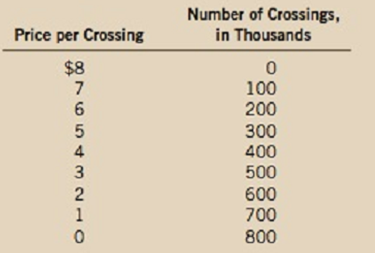Chapter 15, Problem 4PA

Chapter
Section
Textbook Problem

A company is considering building a bridge across a river. The bridge would cost $2 million to build and nothing to maintain. The following table shows the company's anticipated demand over the lifetime of the bridge:a. If the company were to build the bridge, what would be its profit-maximizing price? Would that be the efficient level of output? Why or why not?b. If the company is interested in maximizing profit, should it build the bridge? What would be its profit or loss?c. If the government were to build the bridge, what price should it charge?d. Should the government build the bridge? Explain. Subpart (a): To determine Measuringrevenues, costs and profits. Explanation Total revenue can be calculated by using the following formula. Total Revenue=Price × Quantity (1) Substitute the respective values in Equation (1) to calculate the total revenue at quantity 100 units. Total revenue=7×100=700 Total revenue is$700.

Marginal revenue can be calculated as follows:

Marginal revenue=Total revenuePresentTotal revenuePreviousQuantityPresentQuantityPrevious (2)

Substitute the respective values in Equation (2) to calculate the marginal revenue at quantity 100 units.

Marginal revenue=70001000=7

Marginal revenue is \$7

Subpart (b):

To determine
Measuringrevenues, costs and profits.

Subpart (c):

To determine
Measuringrevenues, costs and profits.

Subpart (d):

To determine
Measuringrevenues, costs and profits.

Still sussing out bartleby?

Check out a sample textbook solution.

See a sample solution

The Solution to Your Study Problems

Bartleby provides explanations to thousands of textbook problems written by our experts, many with advanced degrees!

Get Started